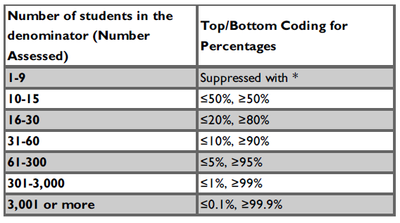# App Development

Announcements
QlikWorld 2023, a live, in-person thrill ride. Save \$300 before February 6: REGISTER NOW!
cancel
Showing results for
Did you mean:Partner - Creator III

## Any advice on reducing this measure expression?

Hello,

I am working on a report that has some fairly restricting PII suppression rules for student data. Basically, given a group of students, I am calculating the percentage of those students that fall into a subgroup using the following table:So if my overall group of students is 35 and the percentage of students in the subgroup is 5%, I show the value <=5%. But if the percentage of students is 15%, then I can show the value of 15% in the report. It essentially boils down to, figure out the number of students in the group to determine what rules to use for suppressing the data. Unfortunately, it ends up being a ton of IF statements:

``````=if(count({\$} [Student]) < 10,
'*',
if(count({\$} [Student]) < 16,
if(count({\$<[Level]={1}>}[Student])/count({\$}[Student])<=0.5, '<=50%',
if(count({\$<[Level]={1}>}[Student])/count({\$}[Student])>=0.5, '>=50%',
count({\$<[Level]={1}>}[Student])/count({\$}[Student])
)),
if(count({\$} [Student]) < 31,
if(count({\$<[Level]={1}>}[Student])/count({\$}[Student])<=0.2, '<=20%',
if(count({\$<[Level]={1}>}[Student])/count({\$}[Student])>=0.8, '>=80%',
count({\$<[Level]={1}>}[Student])/count({\$}[Student])
)),
if(count({\$} [Student]) < 61,
if(count({\$<[Level]={1}>}[Student])/count({\$}[Student])<=0.1, '<=10%',
if(count({\$<[Level]={1}>}[Student])/count({\$}[Student])>=0.9, '>=90%',
count({\$<[Level]={1}>}[Student])/count({\$}[Student])
)),
if(count({\$} [Student]) < 301,
if(count({\$<[Level]={1}>}[Student])/count({\$}[Student])<=0.05, '<=5%',
if(count({\$<[Level]={1}>}[Student])/count({\$}[Student])>=0.95, '>=95%',
count({\$<[Level]={1}>}[Student])/count({\$}[Student])
)),
if(count({\$} [Student]) < 3001,
if(count({\$<[Level]={1}>}[Student])/count({\$}[Student])<=0.01, '<=1%',
if(count({\$<[Level]={1}>}[Student])/count({\$}[Student])>=0.99, '>=99%',
count({\$<[Level]={1}>}[Student])/count({\$}[Student])
)),
if(count({\$<[Level]={1}>}[Student])/count({\$}[Student])<=0.001, '<=0.1%',
if(count({\$<[Level]={1}>}[Student])/count({\$}[Student])>=0.999,'>=99.9%',
count({\$<[Level]={1}>}[Student])/count({\$}[Student])
))
))))))``````

Unfortunately, this is the simplified version of the expression. The actual expression has a few more rules in calculating the values. I believe the number of count functions is around 115, and this is just for one measure. Needless to say, I am worried about performance degradation due to these complex expressions.

Normally when I have a lot of IF statements, I try to use pick(match()) to improve the performance, but I don't think I can apply that here because the conditions are based on a range of values. Is there any way to improve this expression so it doesn't have to calculate every single count function?

0 RepliesTags
Community Browser